## 请选出正确答案，共10题，每题10分，满分100分：

1. Given: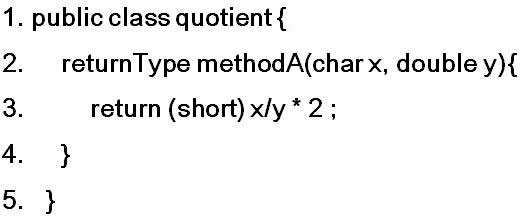What is the valid returnType for methodA in line 2?

A. double

B. int

C. long

D. short

E. char

2.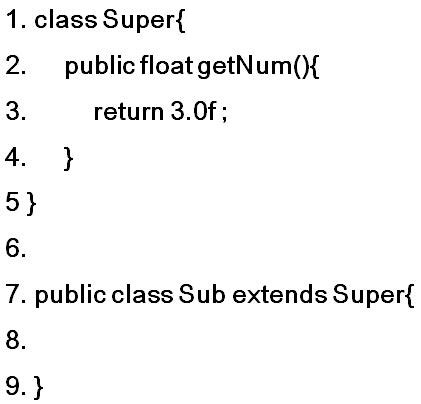which method, placed at line 8, will cause a compiler error?

A. public float getNum(){return 4.0f;}

B. public void getNum(){}

C. public void getNum(double d){}

D. public double getNum(float d){return 4.0d;}

E. private double getNum(){return 4.0d;}

3. Which of the following will evaluate to true only if boolean expressions A, B, and C are all false?

A. !A && !(B && !C)

B. !A || !B || !C

C. !(A || B || C)

D. !A &&!(B || !C)

E. !(A && B && C)

4. Which of the following program fragments will produce this output? (Ignore spacing.)
2 - - - - -
- 4 - - - -
- - 6 - - -
- - - 8 - -
- - - - 10 -
- - - - - 12

III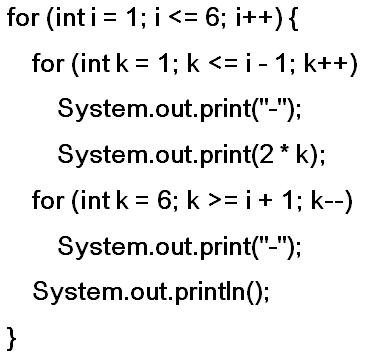III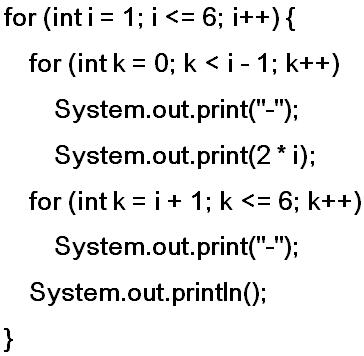A. II only

B. I and II only

C. II and III only

D. I and III only

E. I, II, and III

5. In the following code segment, you may assume that a, b, and n are all type int.A. /* statement 1 */ will be executed.

B. /* statement 2 */ will be executed.

C. Either /* statement 1 */ or /* statement 2 */ will be executed.

D. A compile-time error will occur.

E. A compile-time error will occur.

6. Which statement about parameters is false?

A. The scope of parameters is the method in which they are defined.

B. Static methods have no implicit parameter this.

C. Two overloaded methods in the same class must have parameters with different names.

D. All parameters in Java are passed by value.

E. Two different constructors in a given class can have the same number of parameters.

7. In the following code, the constructors in the Rational class allow initialization of Rational objects in several different ways. Which of the following will cause an error?
public class Rational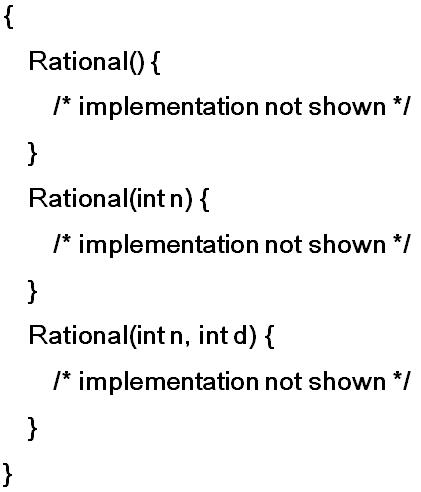A. Rational r1 = new Rational();

B. Rational r2 = r1;

C. Rational r3 = new Rational(2,-3);

D. Rational r4 = new Rational(3.5);

E. Rational r5 = new Rational(10);

8. int index=1;
int foo[]=new int;
int bar=foo[index];
int baz=bar+index;

what is the result?

A. baz has a value of 0

B. baz has value of 1

C. baz has value of 2

D. an exception is thrown

E. the code will not compile

9. If you have the following classes. Which of the following constructors would be valid for Point3D?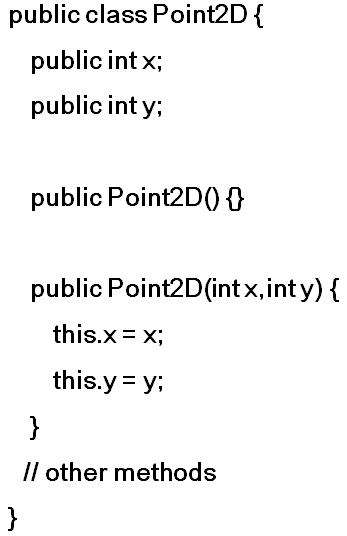I.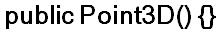II.III.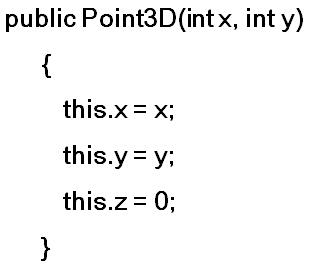A. I only

B. II only

C. III only

D. I and II only

E. I, II, and III

10. Assume that Base b = new Derived(); appears in a client program. What is the result of the call b.methodOne();?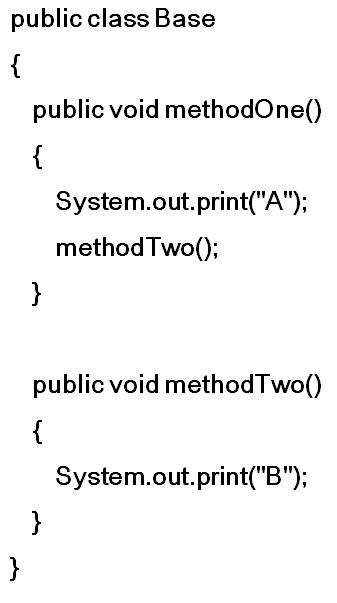A. ABDC

B. AB

C. ABCD

D. ABC

E. ADC• 获取验证码